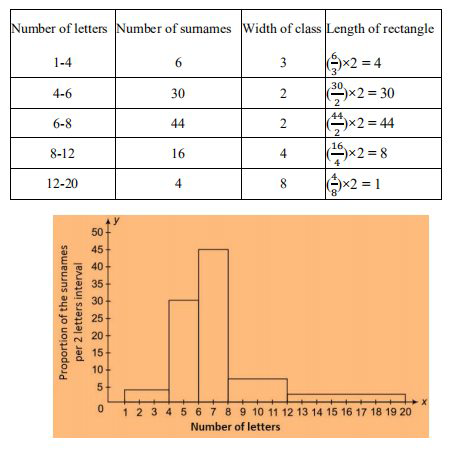# NCERT Solutions Class 9 Mathematics Solutions for Statistics - Exercise 14.3 in Chapter 14 - Statistics

Question 9 Statistics - Exercise 14.3

100 surnames were randomly picked up from a local telephone directory and a frequency distribution of the

number of letters in the English alphabet in the surnames was found as follows:(i) Draw a histogram to depict the given information.

(ii) Write the class interval in which the maximum number of surnames lie.

(i) The width of the class intervals in the given data is varying.

We know that,

the area of the rectangle is proportional to the frequencies in the histogram.

Thus, the proportion of the number of surnames per 2 letters interval can be calculated as given in the table

below.(ii) 6-8 is the class interval in which the maximum number of surnames lie.

Related Questions

Lido

Courses

Teachers

Book a Demo with us

Syllabus

Maths
CBSE
Maths
ICSE
Science
CBSE

Science
ICSE
English
CBSE
English
ICSE
Coding

Terms & Policies

Selina Question Bank

Maths
Physics
Biology

Allied Question Bank

Chemistry
Connect with us on social media!